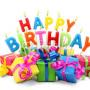"Hey GTQ users! Welcome to my quiz. Do you think that I can guess your birthday. Your answer may be "no". But in this quiz it is possible. Come on and take this quiz"

"You will find this quiz interesting. It is a quiz to predict your birthday. This is really an interesting quiz and you will not find any other quizzes like this. Come on!"

Created by: amazon

1. Take the number of month in which you were born.( January=1,February=2 and so on)[Example: If you were born in June then take the number as 6]
2. Multiply the number by 2.(Example:If your number was 6 then 6*2=12)
4. Multiply the number by 5. (Example: If your answer was 17 then 17*5=85)